Registrations for the November 2021 System Design Cohort are now open# Deciphering Single-byte XOR Ciphertext

Published on 21st Jun 2020

Encryption is a process of encoding messages such that it can only be read and understood by the intended parties. The process of extracting the original message from an encrypted one is called Decryption. Encryption usually scrambles the original message using a key, called encryption key, that the involved parties agree on.

The strength of an encryption algorithm is determined by how hard it would be to extract the original message without knowing the encryption key. Usually, this depends on the number of bits in the key - bigger the key, the longer it takes to decrypt the enciphered data.

In this essay, we will work with a very simple cipher (encryption algorithm) that uses an encryption key with a size of one byte, and try to decipher the ciphered text and retrieve the original message without knowing the encryption key. The problem statement, defined above, is based on Cryptopals Set 1 Challenge 3.

# Single-byte XOR cipher

The Single-byte XOR cipher algorithm works with an encryption key of size 1 byte - which means the encryption key could be one of the possible 256 values of a byte. Now we take a detailed look at how the encryption and decryption processes look like for this cipher.

## Encryption

As part of the encryption process, the original message is iterated bytewise and every single byte `b` is XORed with the encryption key `key` and the resultant stream of bytes is again translated back as characters and sent to the other party. These encrypted bytes need not be among the usual printable characters and should ideally be interpreted as a stream of bytes. Following is the python-based implementation of the encryption process.

``````def single_byte_xor(text: bytes, key: int) -> bytes:
"""Given a plain text `text` as bytes and an encryption key `key` as a byte
in range [0, 256) the function encrypts the text by performing
XOR of all the bytes and the `key` and returns the resultant.
"""
return bytes([b ^ key for b in text])
``````

As an example, we can try to encrypt the plain text - `abcd` - with encryption key `69` and as per the algorithm, we perform XOR bytewise on the given plain text. For character `a`, the byte i.e. ASCII value is `97` which when XORed with `69` results in `36` whose character equivalent is `\$`, similarly for `b` the encrypted byte is `'`, for `c` it is `&` and for `d` it is `!`. Hence when `abcd` is encrypted using single-byte XOR cipher and encryption key `69`, the resultant ciphertext i.e. the encrypted message is `\$'&!`.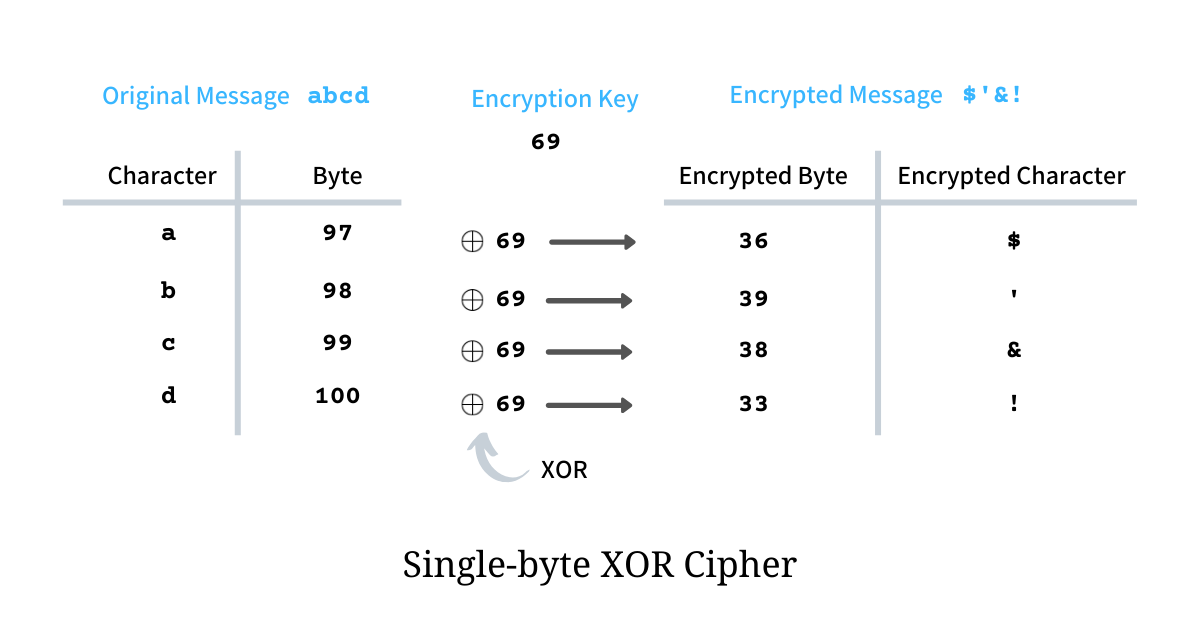## Decryption

Decryption is the process of extracting the original message from the encrypted ciphertext given the encryption key. XOR has a property - if `a = b ^ c` then `b = a ^ c`, hence the decryption process is exactly the same as the encryption i.e. we iterate through the encrypted message bytewise and XOR each byte with the encryption key - the resultant will be the original message.

Since encryption and decryption both have an exact same implementation - we pass the ciphertext to the function `single_byte_xor`, defined above, to get the original message back.

``````>>> single_byte_xor(b"\$'&!", 69)
b'abcd'
``````

# Deciphering without the encryption key

Things become really interesting when we have to recover the original message given the ciphertext and having no knowledge of the encryption key; although we do know the encryption algorithm.

As a sample plain text, we take the last couple of messages, sent across on their German military radio network during World War II. These messages were intercepted and decrypted by the British troops. During wartime, the messages were encrypted using Enigma Machine and Alan Turing famously cracked the Enigma Code (similar to encryption key) that was used to encipher German messages.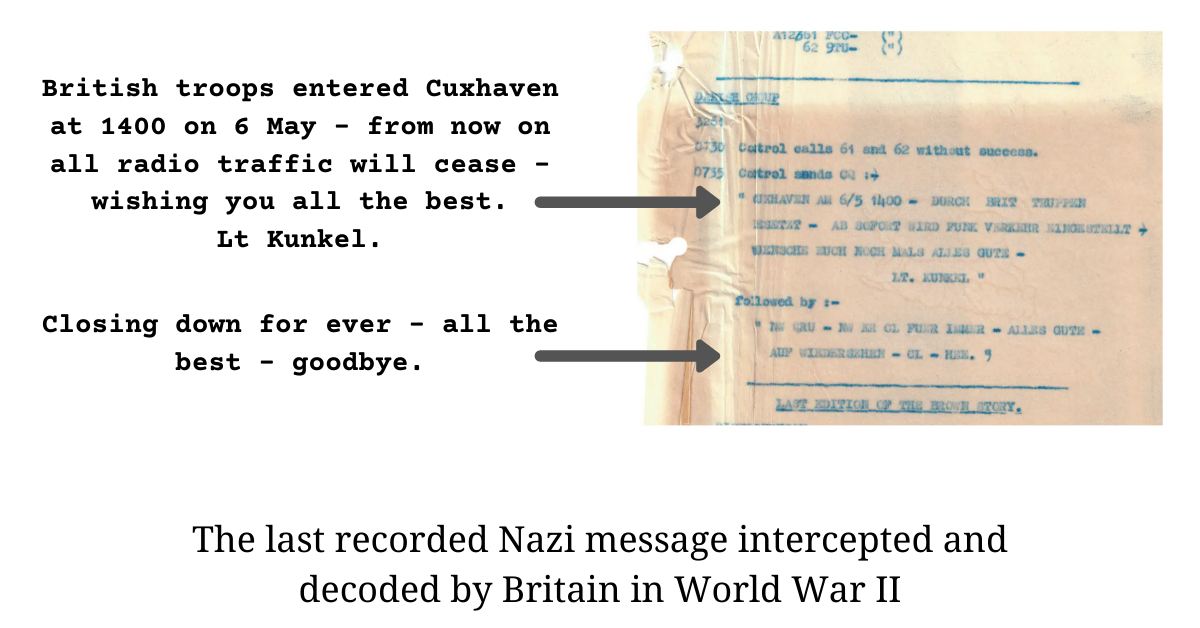In this essay, instead of encrypting the message using the Enigma Code, we are going to use Single-byte XOR cipher and try to recover the original message back without any knowledge of the encryption key.

Here, we assume that the original message, to be encrypted, is a genuine English lowercased sentence. The ciphertext that we would try to decipher can be obtained as

``````>>> key = 82
>>> plain_text = b'british troops entered cuxhaven at 1400 on 6 may - from now on all radio traffic will cease - wishing you all the best. lt kunkel.'
>>> single_byte_xor(plain_text, key)
b'0 ;&;!:r& =="!r7<&7 76r1\'*:3\$7<r3&rcfbbr=<rdr?3+r\x7fr4 =?r<=%r=<r3>>r 36;=r& 344;1r%;>>r173!7r\x7fr%;!:;<5r+=\'r3>>r&:7r07!&|r>&r9\'<97>|'
``````

## Bruteforce

There are a very limited number of possible encryption keys - 256 to be exact - we can, very conveniently, go for the Bruteforce approach and try to decrypt the ciphered text with every single one of it. So we start iterating over all keys in the range `[0, 256)` and decrypt the ciphertext and see which one resembles the original message the most.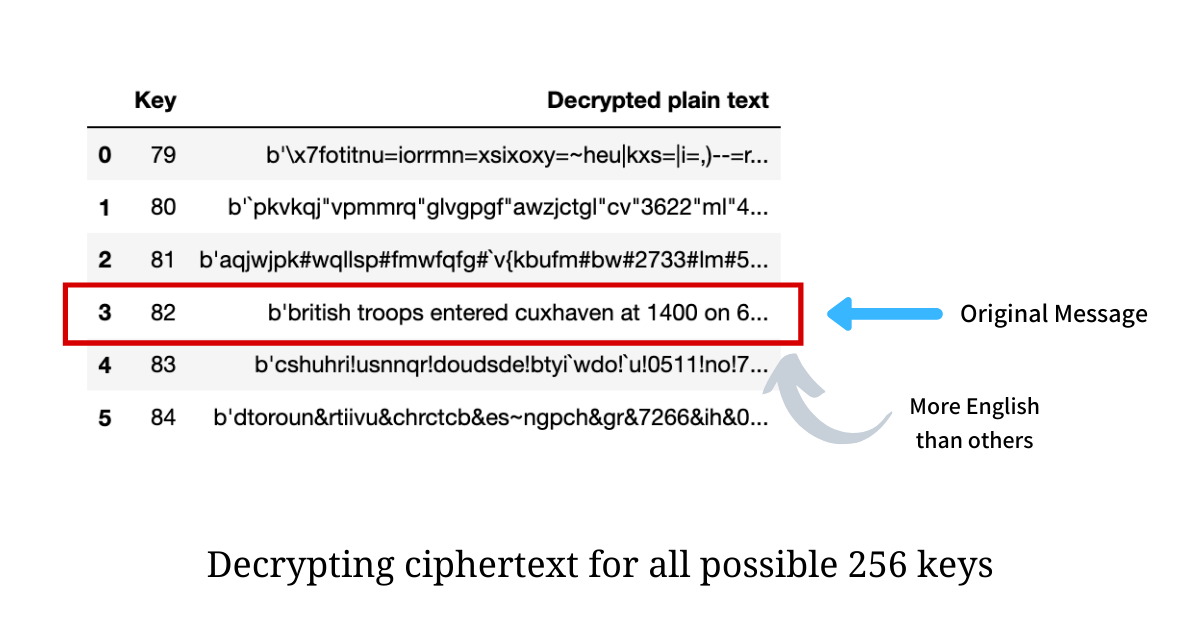In the illustration above, we see that the message decrypted through key `82` is, in fact, our original message, while the other retrieved plain texts look scrambled and garbage. Doing this visually is very easy; we, as humans, are able to comprehend familiarity but how will a computer recognize this?

We need a way to quantify the closeness of a text to a genuine English sentence. Closer the decrypted text is to be a genuine English sentence, the closer it would be to our original plain text.

We can do this only because of our assumption - that the original plain text is a genuine English sentence.

## ETAOIN SHRDLU

Letter Frequency is the number of times letters of an alphabet appear on average in written language. In the English language the letter frequency of letter `a` is `8.239%`, for `b` it is `1.505%` which means out of 100 letters written in English, the letter `a`, on an average, will show up `8.239%` of times while `b` shows up `1.505%` of times. Letter frequency (in percentage) for other letters is as shown below.

``````occurance_english = {
'a': 8.2389258,    'b': 1.5051398,    'c': 2.8065007,    'd': 4.2904556,
'e': 12.813865,    'f': 2.2476217,    'g': 2.0327458,    'h': 6.1476691,
'i': 6.1476691,    'j': 0.1543474,    'k': 0.7787989,    'l': 4.0604477,
'm': 2.4271893,    'n': 6.8084376,    'o': 7.5731132,    'p': 1.9459884,
'q': 0.0958366,    'r': 6.0397268,    's': 6.3827211,    't': 9.1357551,
'u': 2.7822893,    'v': 0.9866131,    'w': 2.3807842,    'x': 0.1513210,
'y': 1.9913847,    'z': 0.0746517
}
``````

This Letter Frequency analysis is a rudimentary way for language identification in which we see if the current letter frequency distribution of a text matches the average letter frequency distribution of the English language. ETAOIN SHRDLU is the approximate order of frequency of the 12 most commonly used letters in the English language.

The following chart shows Letter Frequency analysis for decrypted plain texts with encryption keys from `79` to `84`.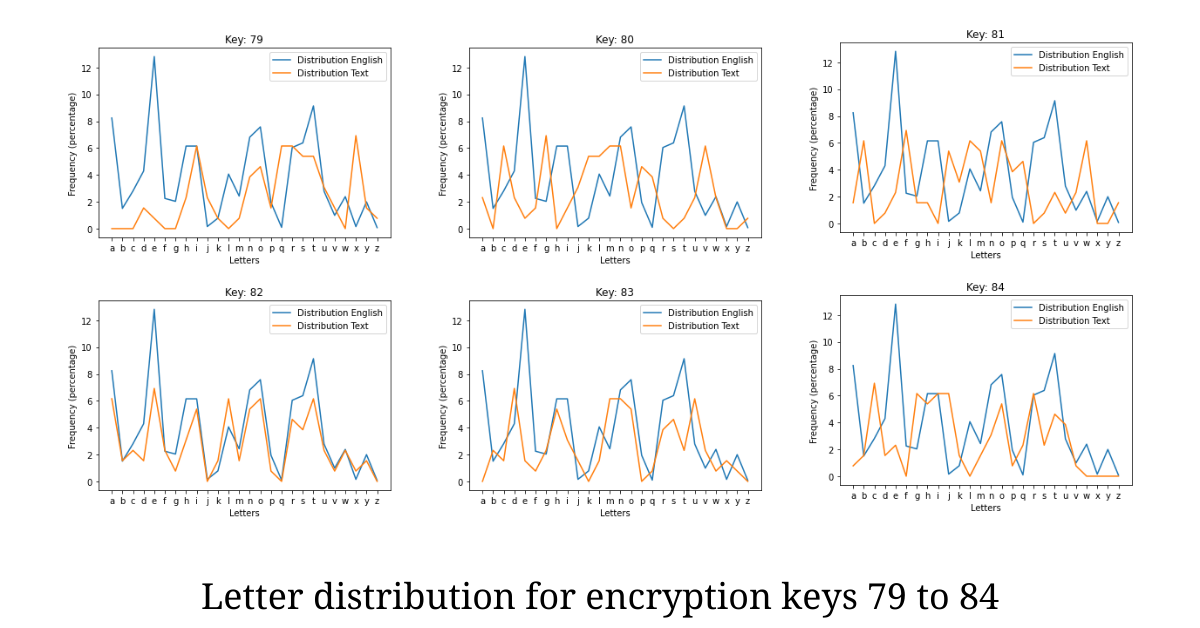In the illustration above, we could clearly see how well the Letter Frequency distribution for encryption key `82` fits the distribution of the English language. Now that our hypothesis holds true, we need a way to quantify this measure and we call if the Fitting Quotient.

## Fitting Quotient

Fitting Quotient is the measure that suggests how well the two Letter Frequency Distributions match. Heuristically, we define the Fitting Quotient as the average of the absolute difference between the frequencies (in percentage) of letters in `text` and the corresponding letter in the English Language. Thus having a smaller value of Fitting Quotient implies the text is closer to the English Language.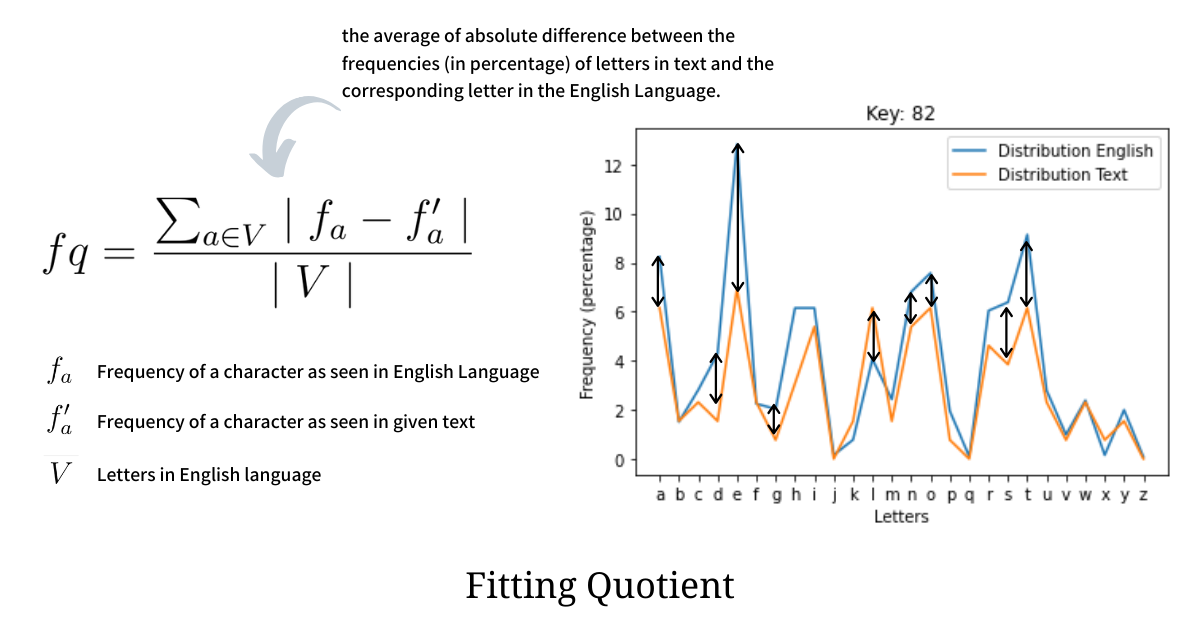Python-based implementation of the, above defined, Fitting Quotient is as shown below. The function first computes the relative frequency for each letter in `text` and then takes an average of the absolute difference between the two distributions.

``````dist_english = list(occurance_english.values())

def compute_fitting_quotient(text: bytes) -> float:
"""Given the stream of bytes `text` the function computes the fitting
quotient of the letter frequency distribution for `text` with the
letter frequency distribution of the English language.

The function returns the average of the absolute difference between the
frequencies (in percentage) of letters in `text` and the corresponding
letter in the English Language.
"""
counter = Counter(text)
dist_text = [
(counter.get(ord(ch), 0) * 100) / len(text)
for ch in occurance_english
]
return sum([abs(a - b) for a, b in zip(dist_english, dist_text)]) / len(dist_text)
``````

## Deciphering

Now that we have everything we require to directly get the plain text out of the given ciphertext we wrap it in a function that iterates over all possible encryption keys in the range `[0, 256)`, decrypts the ciphertext, computes the fitting quotient for the plain text and returns the one that minimizes the quotient as the original message. Python-based implementation of this deciphering logic is as illustrated below.

``````def decipher(text: bytes) -> Tuple[bytes, int]:
"""The function deciphers an encrypted text using Single Byte XOR and returns
the original plain text message and the encryption key.
"""
original_text, encryption_key, min_fq = None, None, None
for k in range(256):
# we generate the plain text using encryption key `k`
_text = single_byte_xor(text, k)

# we compute the fitting quotient for this decrypted plain text
_fq = compute_fitting_quotient(_text)

# if the fitting quotient of this generated plain text is lesser
# than the minimum seen till now `min_fq` we update.
if min_fq is None or _fq < min_fq:
encryption_key, original_text, min_fq = k, _text, _fq

# return the text and key that has the minimum fitting quotient
return original_text, encryption_key
``````

This approach was also tested against 100 random English sentences with random Encryption keys and it was found that this deciphering technique fared well for all the samples. The approach would fail if the sentence is very short or contains a lot of symbols. The source code for this entire deciphering process is available in a Jupyter notebook at arpitbhayani.me/decipher-single-byte-xor.

# References

If my work adds value, consider supporting me## 2000+ Subscribers

If you like what you read subscribe you can always subscribe to my newsletter and get the post delivered straight to your inbox. I write essays on various engineering topics and share it through my weekly newsletter 👇

# Other articles that you might likeDeciphering Repeated-key XOR Ciphertext

Deciphering is the process of recovering the original message from an encrypted byte stream, usually...

4th Jul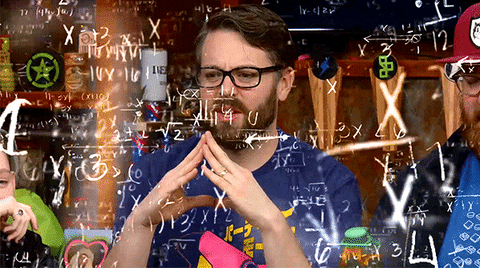Better Ranking using Bayesian Average

Ranking a list of movies, products, books or even restaurants is tricky and in this article, we find...

12th AprIsolation Forest Algorithm for Anomaly Detection

Anomaly detection is an age-old problem and in this article, we dive deep into an unsupervised algor...

28th Jan1D Procedural Terrain Generation

Terrains are at the heart of every Computer Game - be it Counter-Strike, Age of Empires, or even Min...

16th Nov